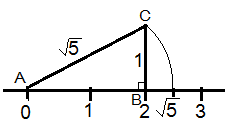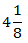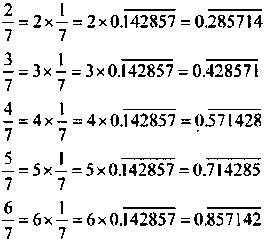# NCERT Solutions for Class 9th Maths: Chapter 1 Number Systems Exercise 1.2 and 1.3

Notes Ncert Solutions Assignments Videos Revision sheet

In this page we have NCERT Solutions for Class 9th Maths: Chapter 1 Number Systems for Exercise 1.2 and 1.3 . Hope you like them and do not forget to like , social share and comment at the end of the page.Free download also available
Question 1.
State whether the following statements are true or false. Justify your answers.

(i) Every irrational number is a real number.
(ii) Every point on the number line is of the form √m, where m is a natural number.
(iii) Every real number is an irrational number.
(i) True, since the collection of real numbers is made up of rational and irrational numbers.
(ii) False, since positive number cannot be expressed as square roots.
(iii) False, as real numbers include both rational and irrational numbers. Therefore, every real number cannot be an irrational number.
Question 2
Are the square roots of all positive integers irrational? If not, give an example of the square root of a number that is a rational number.
No, the square roots of all positive integers are not irrational. For example, √9 = 3.
Question 3
Show how √5 can be represented on the number line.
We can represent √5 using Pythagoras Theorem
What is Pythagoras Theorem
In a right-angled triangle, the square of the hypotenuse is equal to the sum of the squares of the other two sides. Using this theorem, we can represent the irrational numbers on the number line.
Step 1: Let AB be a line of length 2 unit on number line.
Step 2: At B, draw a perpendicular line BC of length 1 unit. Join CA.
Step 3: Now, ABC is a right angled triangle. Applying Pythagoras theorem,
AB2 + BC2 = CA2
⇒ 22 + 12 = CA2
⇒ CA2 = 5
⇒ CA = √5
Thus, CA is a line of length √5 unit.
Step 4: Taking CA as a radius and A as a centre draw an arc touching
the number line. The point at which number line get intersected by
arc is at √5 distance from 0 because it is a radius of the circle
whose centre was A.
Thus, √5 is represented on the number line as shown in the figure.Exercise 1.3
Question 1
Write the following in decimal form and say what kind of decimal expansion each has:
(i) 36/100
(ii) 1/11
(iii)(iv) 3/13
(v) 2/11
(vi) 329/400

 i) 36/100 0.36 (Terminating) ii) 1/11 $0.09090909... = 0.c{09}$ (Non terminating repeating) iii) $4 \frac {1}{8}$ 33/8 = 4.125 (Terminating) iv) 3/13 $0.230769230769... =0.\overline{230769}$(Non terminating repeating) v) 2/11 $0.181818181818... =0.\overline{18}$(Non terminating repeating) vi) 329/400 0.8225 (Terminating)

Question 2
You know that $\frac {1}{7} = 0.\overline{142857}$. Can you predict what the decimal expansion of 2/7, 3/7, 4/7, 5/7, 6/7 are without actually doing the long division? If so, how?
[Hint: Study the remainders while finding the value of 1/7 carefully.]
Yes. We can be done this by:Question 3
Express the following in the form p/q where p and q are integers and q ≠ 0.
i. $.\overline{6}$
ii.$.4 \overline{7}$
iii. $. \overline{001}$
(i) $.\overline{6} = .66666..$
Let x = 0.666...
10x = 6.666...
10x = 6 + x
9x = 6
x = 2/3

(ii) $.4 \overline{7} = .47777..$
= 4/10 + 0.777../10
Let x = 0.777…
10x = 7.777…
10x = 7 + x
x = 7/9
4/10 + 0.777.../10 = 4/10 + 7/90
= 36+7/90 = 43/90
(iii)$. \overline{001} =.001001001...$
Let x = 0.001001...
1000x = 1.001001…
1000x = 1 + x
999x = 1
x = 1/999
Question 4
Let x = 0.9999…
10x = 9.9999…
10x = 9 + x
9x = 9
x = 1
The difference between 1 and 0.999999 is 0.000001 which is negligible. Thus, 0.999 is too much near 1, Therefore, the 1 as answer can be justified.
Question 5
What can the maximum number of digits be in the repeating block of digits in the decimal expansion of 1/17? Perform the division to check your answer.
Answer $\frac {1}{17} = 0.\overline{0588235294117647}$
There are 16 digits in the repeating block of the decimal expansion of 1/17.
Question 6
Look at several examples of rational numbers in the form p/(≠ 0) where p and q are integers with no common factors other than 1 and having terminating decimal representations (expansions). Can you guess what property q must satisfy?
We observe that when q is 2, 4, 5, 8, 10... then the decimal expansion is terminating. For example:
1/2 = 0.5, denominator q = 21
7/8 = 0.875, denominator q = 23
4/5 = 0.8, denominator q = 51

We have observed that terminating decimal may be obtained in the situation where prime factorization of the denominator of the given fractions has the power of 2 only or 5 only or both.

Question 7
Write three numbers whose decimal expansions are non-terminating non-recurring.
Three numbers whose decimal expansions are non-terminating non-recurring are:
0.303003000300003...
0.505005000500005...
0.7207200720007200007200000…
Question 8
Find three different irrational numbers between the rational numbers 5/7 and 9/11.
$\frac {5}{7} = .\overline{714285}$
$\frac {9}{11} = .\overline{81}$
Three different irrational numbers are:
0.740740074000734000074...
0.77077007300077000077....
0.79079007600079000079...
Question 9
Classify the following numbers as rational or irrational:
(i) $\sqrt {23}$
(ii) $\sqrt {225}$
(iii) $0.3796$
(iv) $7.478478....$
(v) $1.101001000100001.....$
(i) $\sqrt {23} = 4.79583152331...$
Since the number is non-terminating non-recurring therefore, it is an irrational number.
(ii)$\sqrt {225} = 15 = 15/1$
Since the number is rational number as it can be represented in p/q form.
(iii)$0.3796$
Since the number is terminating therefore, it is an rational number.
(iv) $7.478478....= 7.\overline{478}$$Since this number is non-terminating recurring, therefore, it is a rational number. (v)$1.101001000100001.....\$
Since the number is non-terminating non-repeating, therefore, it is an irrational number.

Reference Books for class 9 Math

Given below are the links of some of the reference books for class 9 Math.

1. Mathematics for Class 9 by R D Sharma One of the best book for studying class 9 level mathematics. It has lot of problems to be solved.
2. Secondary School Mathematics for Class 9 by R S Aggarwal This is also as good as R.D. Sharma. Either this or the book by R.D. Sharma will do. I find book R.S. Aggarwal little bit more challenging than the one by R.D. Sharma.
3. Pearson IIT Foundation Series - Maths - Class 9 Buy this book if you want to challenge yourself further and want to prepare for JEE foundation.
4. Pearson IIT Foundation Physics, Chemistry & Maths combo for Class 9 Only buy if you are prepared to study extra topics and want to take your studies a step further. You might need help to understand topics in these books.

You can use above books for extra knowledge and practicing different questions.

Note to our visitors :-

Thanks for visiting our website.